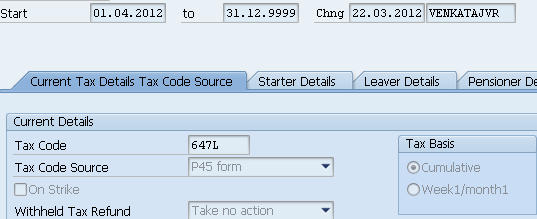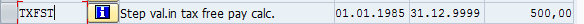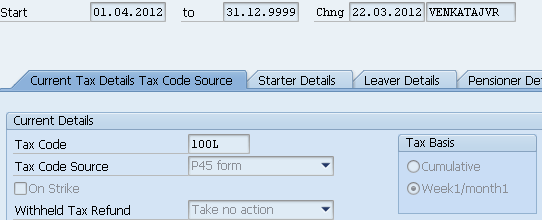# Tax Calculation for UK Payroll

## Purpose

This wiki page explains the calculation logic for tax for UK payroll.

## Overview

Tax calculation for UK payroll is done based on the tax code entered in Infotype 0065 where the logic for calculation depends on the tax code and the tax code basis , for the period for which payroll is run.
The tax calculation logic depends on the following tax code basis
1. Cumulative tax code basis
2. Week1/Month1 tax code basis

## 1.Cummulative Tax code Basis Scenario

Tax calculation logic for an employee with a cumulative tax code basis.
Info type 0065 for the employee is maintained as follows:-Salary of the employee 3000 GBP
Fetching Tax Step Value:From T511k table, the tax step value is fetched which is 500 GBP
Get tax Code Number:
Example: 647 is tax code number for Tax code 647L
Calculating Tax Free Pay:

The tax free pay should be calculated likewise

Tax Free pay = (tax code number – tax step)/12 + (tax step/12)

= (6479 -5000)/12 + 5000/12

= 123.25 + 416.67

= 539.92

Please note that we add 9 to the end of the tax code when calculating the tax free pay.
The same tax free pay can be calculated likewise i.e. for a tax code of  647L , the tax free pay will be 6479/12 = 539.92

Actual Taxable pay = Basic Pay + Total Previous Taxable Pay + P45 Taxable Pay – (Tax Free Pay * total months)
= 3000 + 0 + 0 – (539.92 * 1)
= 2460.08
Tax Free Pay, 539.92 is used to calculate the Actual Taxable Pay
Fetching tax bands:
from table T5G01, tax rate fetched based on paydate, in our example,For 1 month, taxable threshold
= 37400/12 = 3117
Tax calculated:
= (Actual Taxable Pay * 20%) – Tax Paid Previously
= (2460 * 20%) – 0
= 492

## 2.Week1/Month1 tax code basis Scenario

Tax calculation for an employee who is on a week1/month1 basis
Info type 0065 for the employee is maintained as follows:-Salary of the employee 3000 GBP
Fetching Tax Step Value:
From T511k table, the tax step value is fetched which is 500 GBPGet tax Code Number:
Example: 100 is tax code number for Tax code 100L
Calculating Tax Free Pay:
Tax free pay = (tax code number)/12
= 83.33
Actual Taxable pay
= 3000 – 84.09
= 2916.67
Fetching tax bands:
from table T5G01, tax rate fetched based on paydate, in our example,Tax calculated
= Actual Taxable Pay * percentage threshold
= 2915 * 20%
= 583